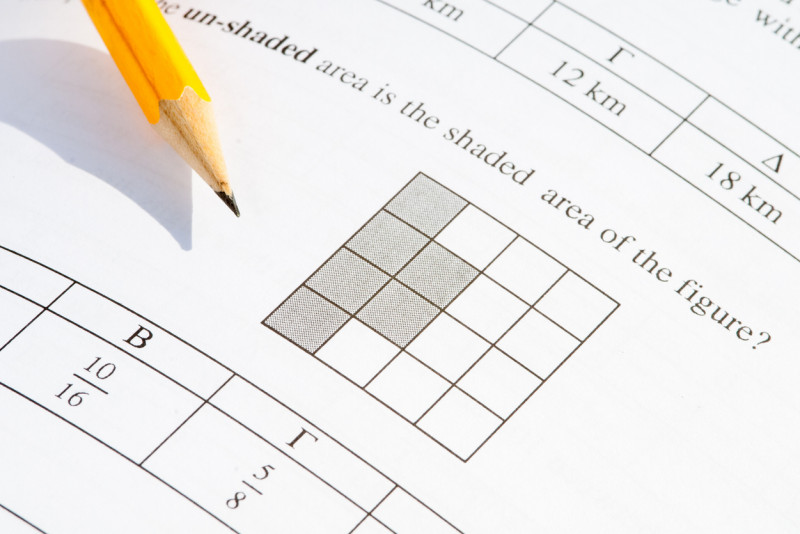# Could You Pass GCSE Maths?

Do you think you could pass a GCSE maths exam today? Test your knowledge with 10 questions from a GCSE foundation maths paper and find out...
Could You Pass GCSE Maths?
By Carli Allan
Do your children attend a Thailand school? Take our survey and help other parents.### Could you pass a GCSE maths exam?

Whether it's been 12 months or 12 years since you last studied maths, how well would you fare if you took your exams again today?

As GCSE students count down to results day next month, we've taken 10 questions from an actual GCSE Maths paper to test your number knowledge.

These questions are taken from AQA’s Foundation Tier Maths (non-calculator) paper from 2022.

1. Circle the answer to 150 ÷ 5.

• 30
• 300
• 3,000

2. Circle the answer to 5 – 7.

• –12
• –2
• 2
• 12

3. Circle the answer to –3 × 3

• –9
• –6
• 6
• 9

4. P is double r. Circle the correct formula.

• P = r / 2
• P = r + 2
• P = r – 2
• P = 2r

5. By rounding each number to the nearest 10, estimate the value of 31 × 18.

6. Work out 60 ÷ 2 + 4.

7.  Cereal: 49p per 100 grams.  Pasta: 14p per 100 grams.

Leah buys 400 grams of cereal and 250 grams of pasta. Work out the total cost in £.

8. For a set of five numbers, the mode is 8, the median is 12. Work out one possible set of five numbers.

9. The term-to-term rule for a sequence is multiply by 2. The 3rd term of the sequence is 46. Work out the 1st term. Give your answer as a decimal.

10. Simplify 8 × 26 × 24. Give your answer as a power of 2.

1. 30
2. –2
3. –9
4. P = 2r
5. 31 × 20, with answer 600
6. 60 ÷ 2 = 30 + 4 = 34
7. £2.31 (0.49 × 4, 0.14 × 2.5)
8. 8 8 12 16 25
9. 46 ÷ 2 = 23. 23 ÷ 2 = 11.5
or
4x = 46. 46 ÷ 4 = 11
10. 213      (26 × 24 =) 26 + 4  or  (26 × 24 =) 210  or  29 (× 24)

Next: Do you have what it takes to pass a higher tier maths GCSE paper?